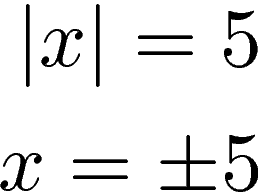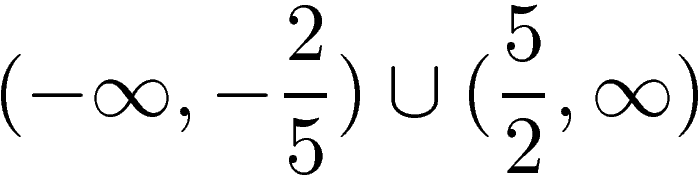# Absolute Value Equations & Inequalities

The absolute value of a number is its distance from 0.  For example, 5 and -5 both have an absolute value of 5 because both are 5 units from 0. The symbols used for absolute value are |  | with a number or variable placed inside the vertical bars. With this knowledge lets look at an example of an absolute value.The answer is +5 because both 5 and -5 are 5 units from 0.

In this post, we will look at equations and inequalities that use absolute values.

Solving one Absolute Value Equations

It is also possible to have inequalities with absolute values. To solve these you want to isolate the absolute value and solve the positive and also the negative version of the answer. Lastly, you never manipulate anything inside the absolute value brackets. you only manipulate and simplify values outside of the brackets. Below is an example.As you can see absolute value inequalities involves solving two equations. Below is an example involving multiplication.Notice again how the values inside the absolute value were never changed. This is important when solving absolute value inequalities.

Solving Two Absolute Values Equations

Solving two absolute values is not that difficult. You simply make one of the absolute values negative for one equation and positive for another. Below is an example.Absolute Value Inequalities

Absolute value inequalities require a slightly different approach. You can rewrite the inequality in double inequality form and solve appropriately when the inequality is “less than.” Below is an example.You can see that we put the absolute value in the middle and simply solved for x. you can even write this using interval notation as shown below.“Greater than” inequalities are solved the same as inequalities with equal signs. You use the “or” concept to solve both inequalities.The interval notation is as followsWe use the union sign in the middle is used in place of the word “or”.

Conclusion

This post provided a brief overview of how to deal with absolute values in both equations and inequalities.